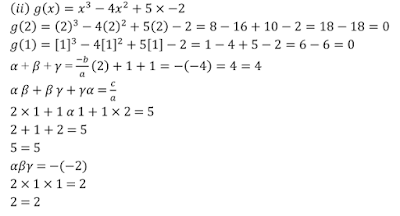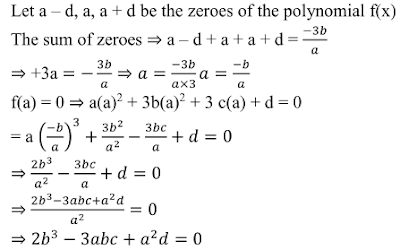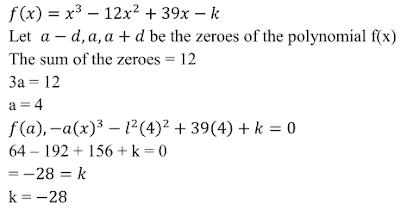## Chapter 2 Polynomials R.D. Sharma Solutions for Class 10th Math Exercise 2.2

Exercise 2.2

1. Verify that the numbers given alongside of the cubic polynomials below are their zeros.
Also, verify the relationship between the zeros and coefficients in each case:
(i) f(x) = 2x3 + x2 - 5x + 2 ; 1/2 , 1, -2
(ii) g(x) = x3 - 4x2 + 5x - 2; 2,1,1

Solution2. Find a cubic polynomial with the sum, sum of the product of its zeroes taken two at a time, and product of its zeros as 3, −1 and −3 respectively.

Solution3. If the zeros of the polynomial f(x) = 2x3 − 15x2 + 37x − 30 are in A.P., find them.

Solution4. Find the condition that the zeros of the polynomial f(x) = x3 + 3px2 + 3qx + r may be in A.P.

Solution5. If the zeroes of the polynomial f(x) = ax3 + 3bx2 + 3cx + d are in A.P., prove that 2b3 - 3abc + a2d = 0

Solution6. If the zeroes of the polynomial f(x) = x3 - 12x2 + 39x + k are in A.P., find the value of k.

Solution